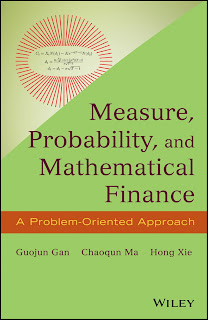ANALYSICS MATHEMATICS

# Measure, Probability, and Mathematical Finance A Problem Oriented Approach Free DownloadProviding both a theoretical and practical approach to the underlying mathematical theory behind financial models, Measure, Probability, and Mathematical Finance: A Problem-Oriented Approach presents important concepts and results in measure theory, probability theory, stochastic processes, and stochastic calculus. Measure theory is indispensable to the rigorous development of probability theory and is also necessary to properly address martingale measures, the change of numeraire theory, and LIBOR market models. In addition, probability theory is presented to facilitate the development of stochastic processes, including martingales and Brownian motions, while stochastic processes and stochastic calculus are discussed to model asset prices and develop derivative pricing models.